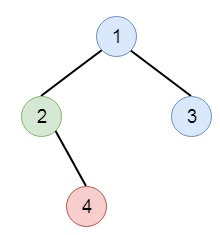# Print Binary Tree in C++

Suppose we have to display a binary tree in an m*n 2D string array based on these rules −

• The row number m should be same as the height of the given binary tree.
• The column number n should be always an odd number.
• The value of the root node should be put in the exactly middle of the first row it can be put. The column and the row where the root node resides, will separate the rest space into two parts. These are left-bottom part and right-bottom part. We should print the left subtree in the left-bottom part and print the right subtree in the right-bottom part. Here the left-bottom part and the right-bottom part should have the same size. Even if one subtree is none while the other is not, we don't need to print anything for the none subtree but still need to leave the space as large as that for the other subtree. Now, if two subtrees are none, then we don't need to leave space for both of them.
• Each unused space should contain an empty string.
• Display the subtrees following the same rules.

So if the input tree is like −Then the output will be −

 1 2 3 4

To solve this, we will follow these steps −

• Define another method called fill(), This will take node, matrix ret, lvl, l and r value
• if node is null, then return
• ret[lvl, (l + r)/2] := node val as string
• fill(left of node, ret, lvl+1, l, (l+r)/2)
• fill(right of node, ret, lvl+1, (l+r+1)/2, r)
• From the main method do following −
• h := height of the tree
• leaves = 2^h – 1
• make a matrix of order h x leaves, and fill this with blank strings
• fill(root, ret, 0, 0, leaves)
• return ret

Let us see the following implementation to get better understanding −

## Example

Live Demo

#include <bits/stdc++.h>
using namespace std;
void print_vector(vector<vector<auto> > v){
cout << "[";
for(int i = 0; i<v.size(); i++){
cout << "[";
for(int j = 0; j <v[i].size(); j++){
cout << v[i][j] << ", ";
}
cout << "],";
}
cout << "]"<<endl;
}
class TreeNode{
public:
int val;
TreeNode *left, *right;
TreeNode(int data){
val = data;
left = right = NULL;
}
};
void insert(TreeNode **root, int val){
queue<TreeNode*> q;
q.push(*root);
while(q.size()){
TreeNode *temp = q.front();
q.pop();
if(!temp->left){
if(val != NULL)
temp->left = new TreeNode(val);
else
temp->left = new TreeNode(0);
return;
} else {
q.push(temp->left);
}
if(!temp->right){
if(val != NULL)
temp->right = new TreeNode(val);
else
temp->right = new TreeNode(0);
return;
} else {
q.push(temp->right);
}
}
}
TreeNode *make_tree(vector<int> v){
TreeNode *root = new TreeNode(v);
for(int i = 1; i<v.size(); i++){
insert(&root, v[i]);
}
return root;
}
class Solution {
public:
int getHeight(TreeNode* node){
if(!node)return 0;
return 1 + max(getHeight(node->left), getHeight(node->right));
}
void fill(TreeNode* node, vector<vector<string>>& ret, int lvl, int l, int r){
if(!node || node->val == 0)return;
ret[lvl][(l + r) / 2] = to_string(node->val);
fill(node->left, ret, lvl + 1, l, (l + r) / 2);
fill(node->right, ret, lvl + 1, (l + r + 1) / 2, r);
}
vector<vector<string>> printTree(TreeNode* root) {
int h = getHeight(root);
int leaves = (1 << h) - 1;
vector < vector <string> > ret(h, vector <string>(leaves, ""));
fill(root, ret, 0, 0, leaves);
return ret;
}
};
main(){
vector<int> v = {1,2,3,NULL,4};
Solution ob;
TreeNode *root = make_tree(v);
print_vector(ob.printTree(root));
}

## Input

[1,2,3,null,4]

## Output

[[, , , 1, , , , ],
[, 2, , , , 3, , ],
[, , 4, , , , , ],]